# 二、检测边缘并应用图像过滤器

• 什么是 2D 卷积以及如何使用
• 如何模糊图像
• 如何检测图像边缘
• 如何将运动模糊应用于图像
• 如何锐化和浮雕图像
• 如何腐蚀和扩大图像
• 如何创建晕影过滤器
• 如何增强图像对比度

# 2D 卷积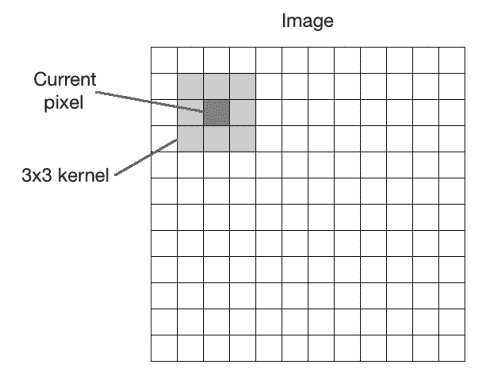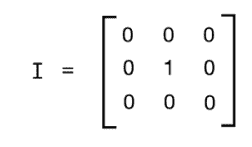# 模糊化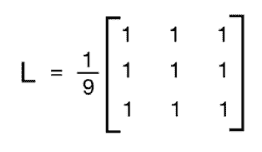import cv2
import numpy as np

rows, cols = img.shape[:2]

kernel_identity = np.array([[0,0,0], [0,1,0], [0,0,0]])
kernel_3x3 = np.ones((3,3), np.float32) / 9.0 # Divide by 9 to normalize the kernel
kernel_5x5 = np.ones((5,5), np.float32) / 25.0 # Divide by 25 to normalize the kernel

cv2.imshow('Original', img)

# value -1 is to maintain source image depth
output = cv2.filter2D(img, -1, kernel_identity) cv2.imshow('Identity filter', output)

output = cv2.filter2D(img, -1, kernel_3x3)
cv2.imshow('3x3 filter', output)

output = cv2.filter2D(img, -1, kernel_5x5)
cv2.imshow('5x5 filter', output)

cv2.waitKey(0)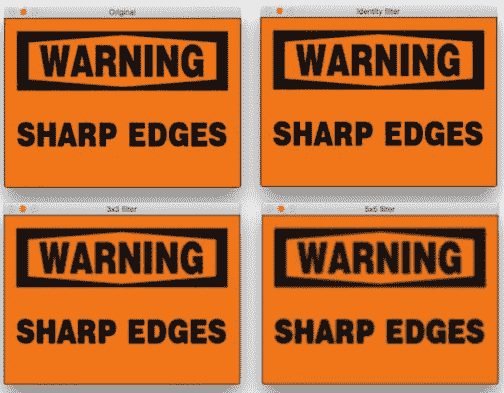# 核大小与模糊

output = cv2.blur(img, (3,3))


# 运动模糊import cv2
import numpy as np

cv2.imshow('Original', img)

size = 15

# generating the kernel
kernel_motion_blur = np.zeros((size, size))
kernel_motion_blur[int((size-1)/2), :] = np.ones(size)
kernel_motion_blur = kernel_motion_blur / size

# applying the kernel to the input image
output = cv2.filter2D(img, -1, kernel_motion_blur)

cv2.imshow('Motion Blur', output)
cv2.waitKey(0)


# 底层原理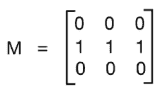# 锐化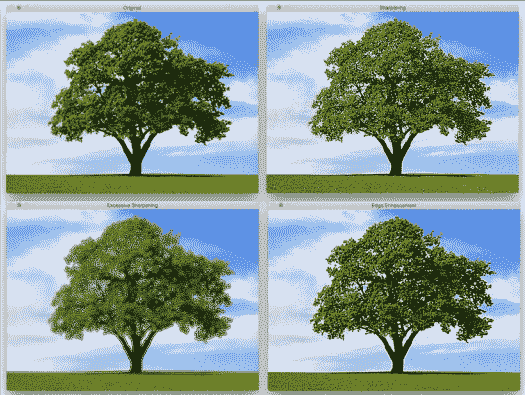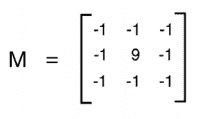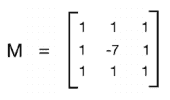import cv2
import numpy as np

cv2.imshow('Original', img)

# generating the kernels
kernel_sharpen_1 = np.array([[-1,-1,-1], [-1,9,-1], [-1,-1,-1]])
kernel_sharpen_2 = np.array([[1,1,1], [1,-7,1], [1,1,1]])
kernel_sharpen_3 = np.array([[-1,-1,-1,-1,-1],
[-1,2,2,2,-1],
[-1,2,8,2,-1],
[-1,2,2,2,-1],
[-1,-1,-1,-1,-1]]) / 8.0

# applying different kernels to the input image
output_1 = cv2.filter2D(img, -1, kernel_sharpen_1)
output_2 = cv2.filter2D(img, -1, kernel_sharpen_2)
output_3 = cv2.filter2D(img, -1, kernel_sharpen_3)

cv2.imshow('Sharpening', output_1)
cv2.imshow('Excessive Sharpening', output_2)
cv2.imshow('Edge Enhancement', output_3)
cv2.waitKey(0)


# 浮雕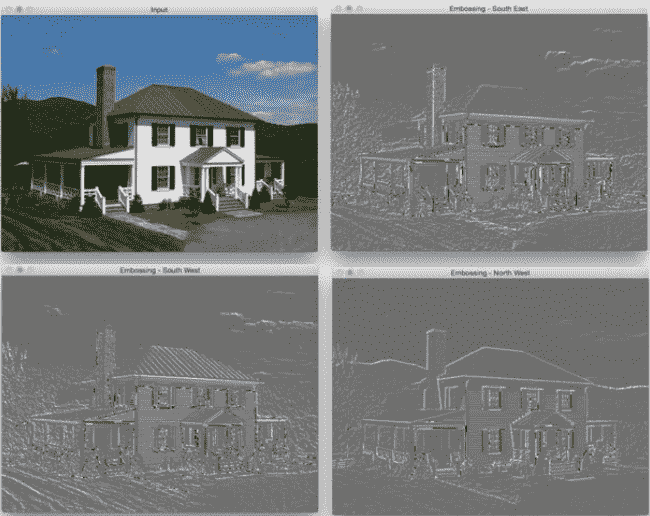import cv2
import numpy as np

# generating the kernels
kernel_emboss_1 = np.array([[0,-1,-1],
[1,0,-1],
[1,1,0]])
kernel_emboss_2 = np.array([[-1,-1,0],
[-1,0,1],
[0,1,1]])
kernel_emboss_3 = np.array([[1,0,0],
[0,0,0],
[0,0,-1]])

# converting the image to grayscale
gray_img = cv2.cvtColor(img_emboss_input,cv2.COLOR_BGR2GRAY)

# applying the kernels to the grayscale image and adding the offset to produce the shadow
output_1 = cv2.filter2D(gray_img, -1, kernel_emboss_1) + 128
output_2 = cv2.filter2D(gray_img, -1, kernel_emboss_2) + 128
output_3 = cv2.filter2D(gray_img, -1, kernel_emboss_3) + 128

cv2.imshow('Input', img_emboss_input)
cv2.imshow('Embossing - South West', output_1)
cv2.imshow('Embossing - South East', output_2)
cv2.imshow('Embossing - North West', output_3)
cv2.waitKey(0)


# 边缘检测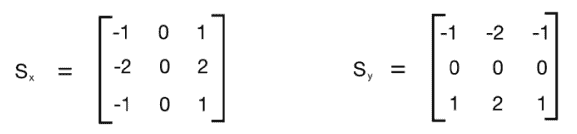import cv2
import numpy as np

rows, cols = img.shape

# It is used depth of cv2.CV_64F.
sobel_horizontal = cv2.Sobel(img, cv2.CV_64F, 1, 0, ksize=5)

# Kernel size can be: 1,3,5 or 7.
sobel_vertical = cv2.Sobel(img, cv2.CV_64F, 0, 1, ksize=5)

cv2.imshow('Original', img)
cv2.imshow('Sobel horizontal', sobel_horizontal)
cv2.imshow('Sobel vertical', sobel_vertical)

cv2.waitKey(0)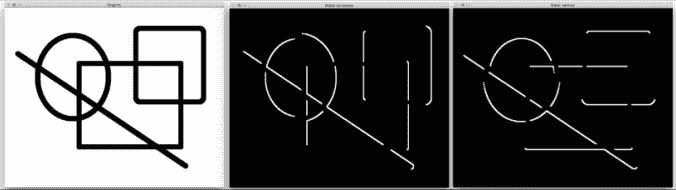laplacian = cv2.Laplacian(img, cv2.CV_64F)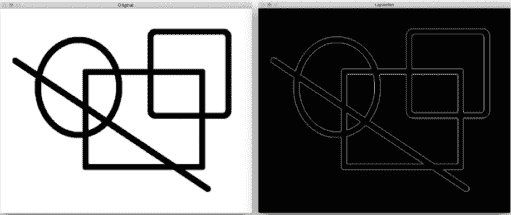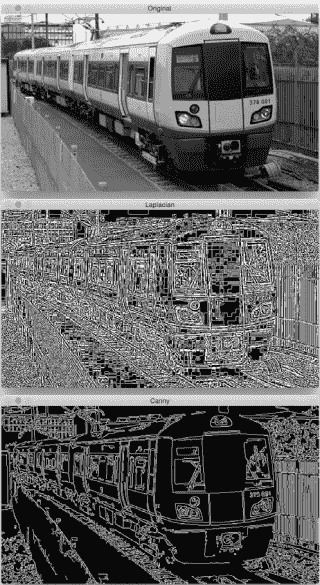canny = cv2.Canny(img, 50, 240)


# 侵蚀和膨胀import cv2
import numpy as np

kernel = np.ones((5,5), np.uint8)

img_erosion = cv2.erode(img, kernel, iterations=1)
img_dilation = cv2.dilate(img, kernel, iterations=1)

cv2.imshow('Input', img)
cv2.imshow('Erosion', img_erosion)
cv2.imshow('Dilation', img_dilation)

cv2.waitKey(0)


# 事后思考

OpenCV 提供直接腐蚀和扩大图像的函数。 它们分别称为腐蚀和膨胀。 值得注意的是这两个函数中的第三个参数。 迭代次数将确定您要腐蚀/扩大给定图像的数量。 它基本上将操作顺序地应用于所得图像。 您可以拍摄样本图像，并使用此参数来查看结果。

# 创建晕影过滤器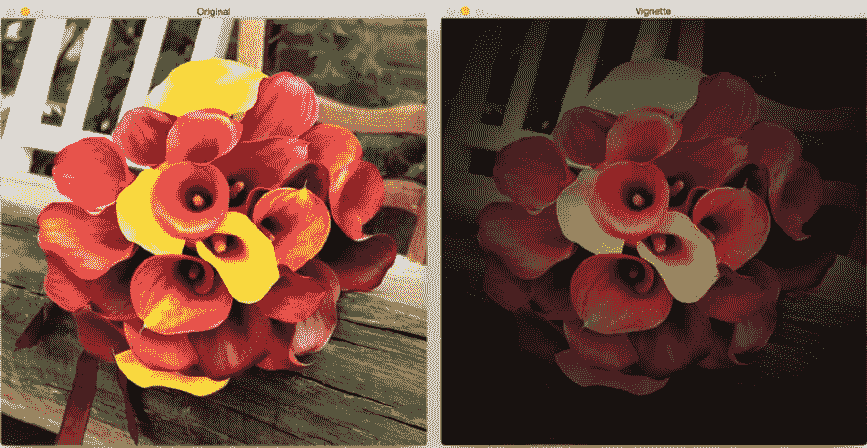import cv2
import numpy as np

rows, cols = img.shape[:2]

# generating vignette mask using Gaussian kernels
kernel_x = cv2.getGaussianKernel(cols,200)
kernel_y = cv2.getGaussianKernel(rows,200)
kernel = kernel_y * kernel_x.T
mask = 255 * kernel / np.linalg.norm(kernel)
output = np.copy(img)

# applying the mask to each channel in the input image
for i in range(3):

cv2.imshow('Original', img)
cv2.imshow('Vignette', output)
cv2.waitKey(0)


# 到底发生了什么？

 mask = 255 * kernel / np.linalg.norm(kernel)


# 我们如何转移焦点？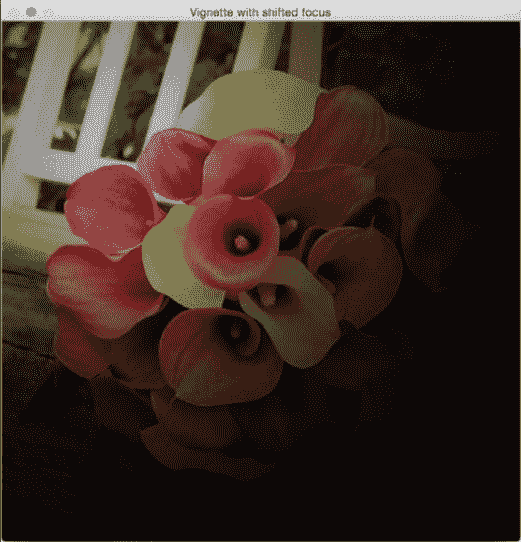import cv2
import numpy as np

rows, cols = img.shape[:2]

# generating vignette mask using Gaussian kernels
kernel_x = cv2.getGaussianKernel(int(1.5*cols),200)
kernel_y = cv2.getGaussianKernel(int(1.5*rows),200)
kernel = kernel_y * kernel_x.T
mask = 255 * kernel / np.linalg.norm(kernel)
output = np.copy(img)

# applying the mask to each channel in the input image
for i in range(3):

cv2.imshow('Input', img)
cv2.imshow('Vignette with shifted focus', output)

cv2.waitKey(0)


# 增强图像的对比度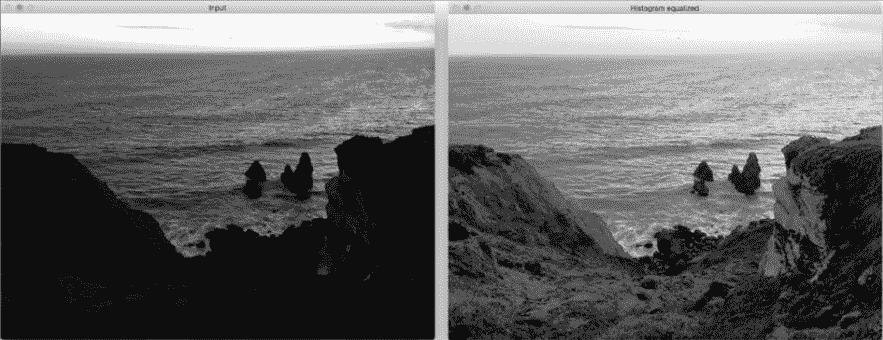import cv2
import numpy as np

# equalize the histogram of the input image
histeq = cv2.equalizeHist(img)

cv2.imshow('Input', img)
cv2.imshow('Histogram equalized', histeq)
cv2.waitKey(0)


# 我们如何处理彩色图像？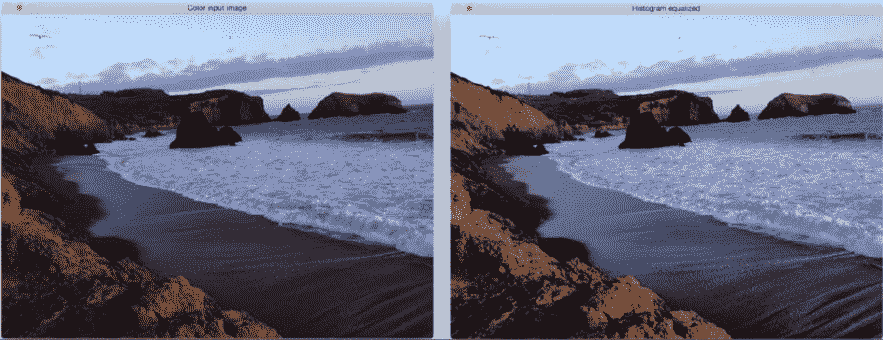import cv2
import numpy as np

img_yuv = cv2.cvtColor(img, cv2.COLOR_BGR2YUV)

# equalize the histogram of the Y channel
img_yuv[:,:,0] = cv2.equalizeHist(img_yuv[:,:,0])

# convert the YUV image back to RGB format
img_output = cv2.cvtColor(img_yuv, cv2.COLOR_YUV2BGR)

cv2.imshow('Color input image', img)
cv2.imshow('Histogram equalized', img_output)

cv2.waitKey(0)